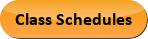Aug 09, 2022
2022-2023 College Catalog
 Select a Catalog 2022-2023 College Catalog 2021-2022 College Catalog [ARCHIVED CATALOG] 2020-2021 College Catalog [ARCHIVED CATALOG] 2019-2020 College Catalog [ARCHIVED CATALOG]
 HELP 2022-2023 College Catalog Print-Friendly Page (opens a new window)

# ENG 220 - Engineering Mechanics: Dynamics

3 Credits, 3 Contact Hours
3 lecture periods 0 lab periods

Study of the motion of bodies under the action of forces. Includes introduction to dynamics, kinematics of particles and rigid body, and kinetics of particles and rigid body.

Prerequisite(s): ENG 210  and MAT 241Course Learning Outcomes
1. Demonstrate mastery to determine the kinematic relationships between positions, velocity, and acceleration for two-dimensional motion of systems of particles and rigid body.
2. Demonstrate the ability to analyze linear and curvilinear motion of particles.
3. Demonstrate the ability to analyze the two-dimensional motion of particles using: mass-force-acceleration method, work energy methods, and impulse-momentum method.
4. Demonstrate the ability to apply method of relative velocity and relative acceleration to provide motion analysis of rigid body.
5. Demonstrate the ability to apply force-mass-acceleration method for kinetic analysis of rigid bodies in plane motion.
6. Demonstrate the ability to apply equations of motion for rigid bodies undergoing translation, rotation about a fixed axis, and general plane motion.

Performance Objectives:
1. Determine the kinematic relationships between positions, velocity, and acceleration for two-dimensional motion of systems of particles and rigid body.
2. Identify and analyze special cases of rectilinear motion (uniform motion, uniformly accelerated motion).
3. Compute position, velocity, and acceleration of particles in relative motion and dependent relative motion.
4. Analyze curvilinear motion in Cartesian (rectangular) coordinate system.
5. Analyze problems of projectile motion.
6. Analyze curvilinear motion in normal-tangential coordinate system.
7. Analyze curvilinear motion in polar coordinate system.
8. Analyze the two-dimensional motion of particles using: mass-force-acceleration method, work energy method and impulse-momentum method.
9. Classify dynamics problems by the best method of solutions.
10. Apply Newton’s law to obtain equations of two-dimensional motion for dynamic systems.
11. Draw free body and kinetics diagrams for particles.
12. Analyze forces in Cartesian (rectangular), normal-tangential, and polar coordinate systems.
13. Compute linear and angular momentum of a particle.
14. Apply the principle of impulse and momentum to problems of direct and oblique central impact.
15. Analyze rotation of the rigid body about a fixed axis.
16. Analyze the planar rigid body motion by using both absolute and translating frame of reference.
17. Apply vector analysis to solve kinematics problems.
18. Apply method of relative velocity and relative acceleration to provide motion analysis of rigid body.
19. Apply graphical method of instantaneous centers to provide motion analysis of rigid body.
20. Apply relative motion analysis using rotating coordinate systems.
21. Determine the Coriolis acceleration in plane motion.
22. Determine the mass moment of inertia of a body.
23. Draw free body and kinetics diagrams for rigid bodies.
24. Compute angular momentum of a rigid body.
25. Apply force-mass-acceleration method for kinetic analysis of rigid bodies in plane motion.
26. Formulate the equations of motion for rigid bodies undergoing translation, and rotation about a fixed axis, and general plane motion.

Outline:
1. Introduction to Dynamics
1. Application of dynamics
2. Basic concepts
3. Newton’s laws
4. Solving problems in dynamics
5. Appropriate units of measurement for dynamics problems
2. Kinematics of Particles
1. Introduction
2. Rectilinear motion
3. Displacement, velocity, and acceleration
4. Graphical interpretations
5. Curvilinear motion
6. Rectangular coordinates
7. Projectile motion
8. Normal and tangential coordinates
9. Circular motion
10. Polar coordinates
3. Kinetics of Particles
1. Introduction
2. Unconstrained and constrained motion
3. Free-body diagram
4. Mass force acceleration method
5. Work energy method
6. Kinetic energy
7. Potential energy
8. Conservation of energy
9. Impulse-momentum method
10. Linear momentum of a particle
11. Conservation of linear momentum
12. Impact
13. Angular momentum of a particle
14. Conservation of angular momentum
4. Kinematics of Rigid Body
1. Introduction
2. Unconstrained and constrained motion
3. Rectilinear translation
4. Curvilinear translation
5. Rotation about a fixed axis
6. Angular-motion relations
7. Absolute motion
8. Principles of relative motion
9. Method of relative velocity
10. Graphical method of instantaneous centers
11. Method of relative acceleration
12. Motion relative to rotating axes; coriolis acceleration
5. Kinetics of Rigid Body
1. Introduction
2. General equations of motion
3. Unconstrained and constrained motion
4. Translation
5. Fixed-axis rotation
6. General plane motion

Effective Term: